# Release Notes for v0.8.2

## Files Changed

``````71 files changed, 1170 insertions(+), 977 deletions(-)
...
``````

## How bad is panic (performancewise)?

I asked this question on the Rust Users Forum and as a result I introduced four new enums (using the strum crate):

``````use strum::IntoEnumIterator;
use strum_macros::EnumIter;
// src/core/geometry.rs
#[derive(EnumIter, Debug, Copy, Clone)]
#[repr(u8)]
pub enum XYEnum {
X = 0,
Y = 1,
}

#[derive(EnumIter, Debug, Copy, Clone)]
#[repr(u8)]
pub enum MinMaxEnum {
Min = 0,
Max = 1,
}

#[derive(EnumIter, Debug, Copy, Clone)]
#[repr(u8)]
pub enum XYZEnum {
X = 0,
Y = 1,
Z = 2,
}
// src/core/spectrum.rs
#[derive(EnumIter, Debug, Copy, Clone)]
#[repr(u8)]
pub enum RGBEnum {
Red = 0,
Green = 1,
Blue = 2,
}
``````

To iterate over the variants of an `Enum`, you do use the strum crate. The old loop might have looked like this:

``````        for axis in 0..3_usize {
for ch in 0..3_usize {
pdf += self.pdf_sr(ch, r_proj[axis])
* n_local[axis as u8].abs()
* ch_prob
* axis_prob[axis];
}
}
``````

Which gets replaced by:

``````use strum::IntoEnumIterator;
...
for axis in XYZEnum::iter() {
for ch in RGBEnum::iter() {
pdf += self.pdf_sr(ch, r_proj[axis as usize])
* n_local[axis].abs()
* ch_prob
* axis_prob[axis as usize];
}
}
``````

## Issues

There was only one issue resolved for this release, which introduced a naming convention (for `parse_blend_file.rs`) like this:

If the instances (objects with transformation info) are called object_name1, object_name2, etc., the shared mesh should be called object_name, and the material (shared for all objects) should be called object_name1 (notice the trailing one).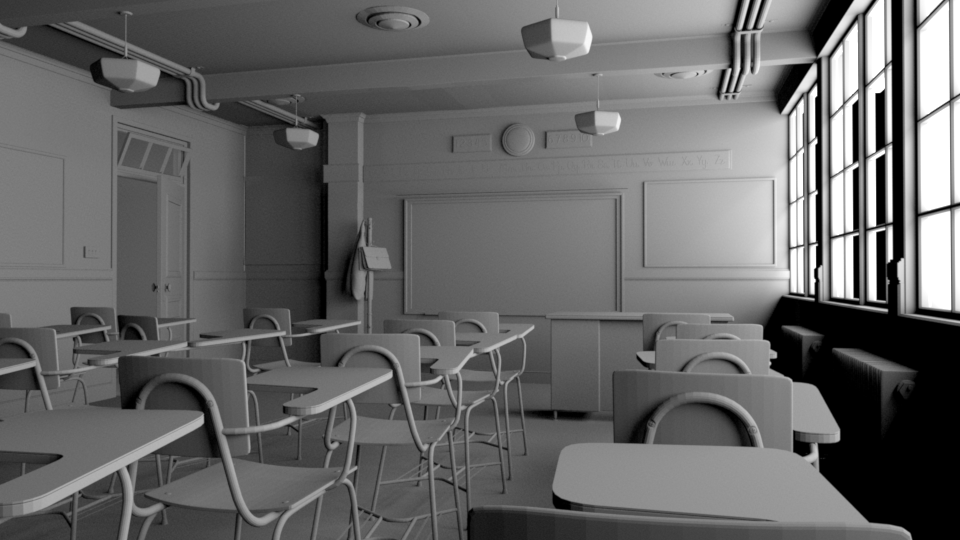This is not using instances on the rendering side, it just allows `.blend` files to share the same mesh geometry for several objects, which place the same geometry several times within the scene.

## Performance

### MediumInterface

Commit 14aa1bc27b9e38a7dc6d905450d335f5679043e7 avoids allocating `MediumInterface` in a loop. The result can be seen via heaptrack: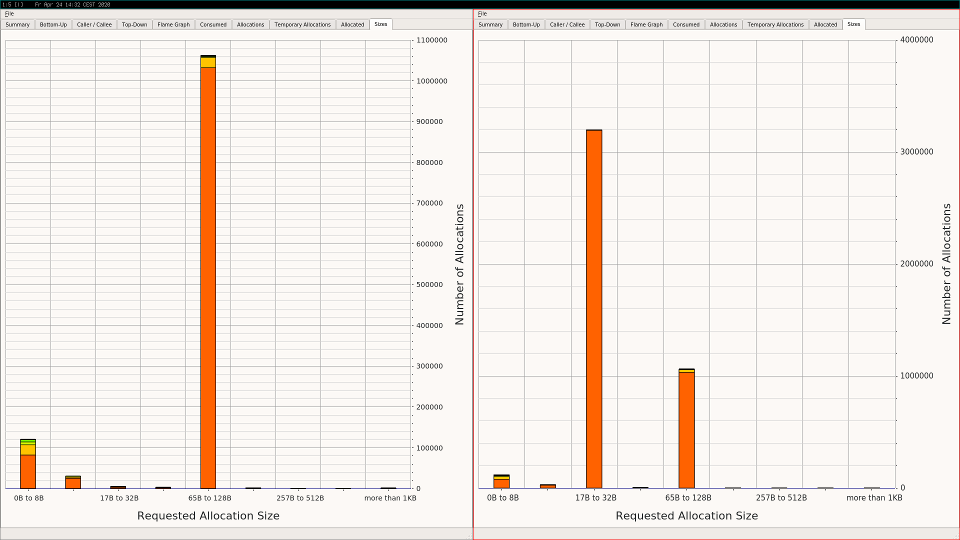The third column on the right side is reduced a lot. All other columns just scale ...

### RwLock

Commit c4858d139fa7da364f48f46854b631ebd4516300 (and the previous commit) replace `RwLock` and the diagrams using perf show this: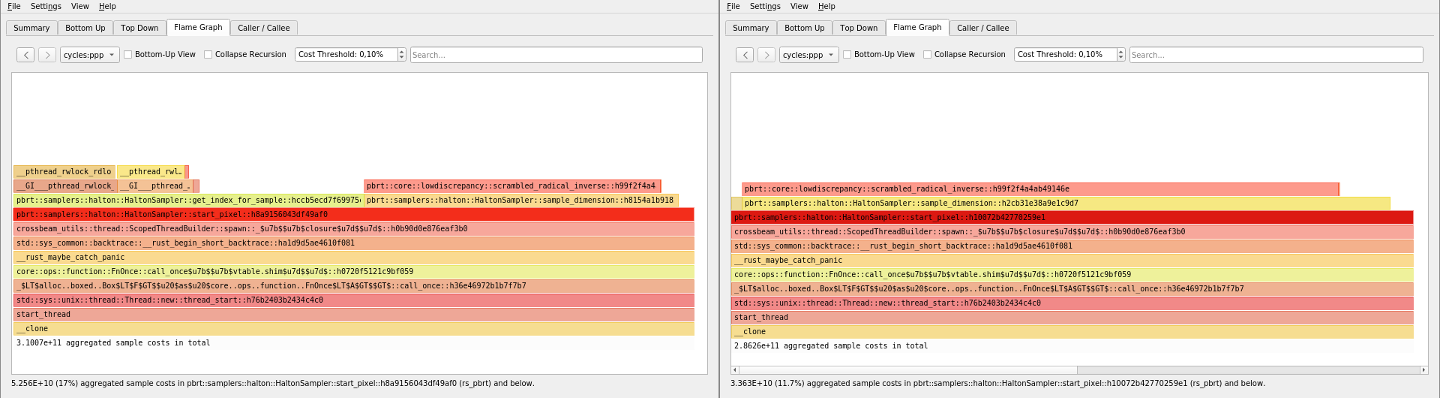``````@@ -54,8 +57,9 @@ pub struct HaltonSampler {
pub base_exponents: Point2i,
pub sample_stride: u64,
pub mult_inverse: [i64; 2],
-    pub pixel_for_offset: RwLock<Point2i>,
-    pub offset_for_current_pixel: RwLock<u64>,
+    pub pixel_for_offset_x: AtomicI32,
+    pub pixel_for_offset_y: AtomicI32,
+    pub offset_for_current_pixel: AtomicU64,
pub sample_at_pixel_center: bool, // default: false
// inherited from class GlobalSampler (see sampler.h)
pub dimension: i64,
``````

Here is another commit which replaces a `RwLock` by an `AtomSetOnce`:

``````@@ -37,10 +38,10 @@ impl LightDistribution {
}
}

-#[derive(Debug, Default)]
+#[derive(Debug)]
struct HashEntry {
packed_pos: Atomic<u64>,
-    distribution: RwLock<Option<Arc<Distribution1D>>>,
+    distribution: AtomSetOnce<Arc<Distribution1D>>,
}

/// The simplest possible implementation of LightDistribution: this
``````

This commit removes memory allocations due to `RwLock`: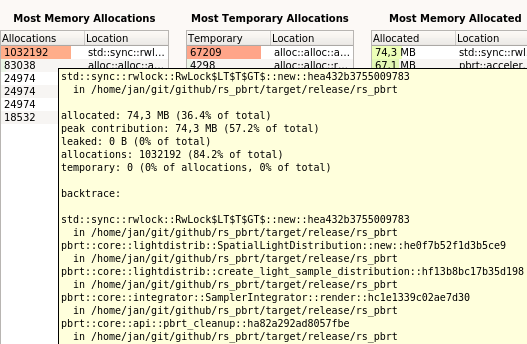On the right you see that the fifth column was pretty high: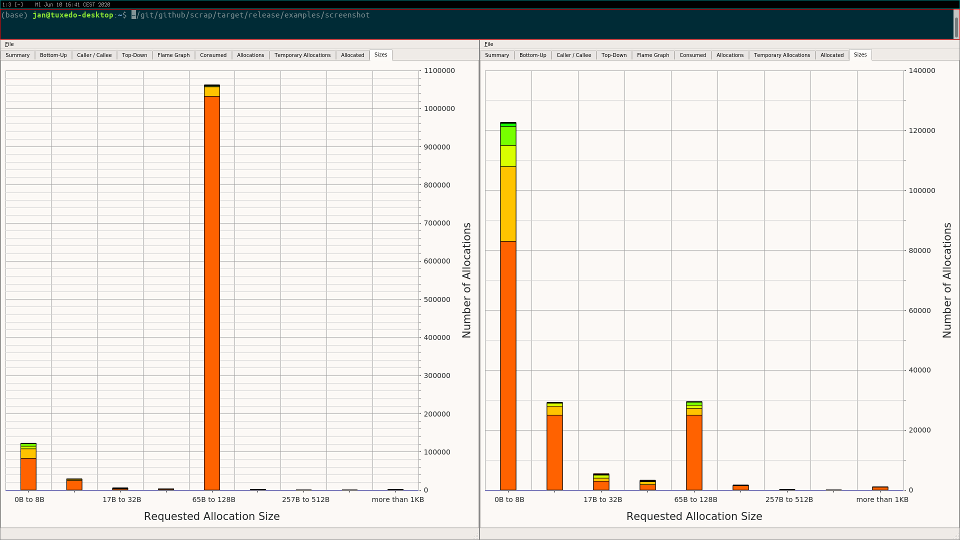Most of the allocations in that row were there because the threads were using `RwLock` in the `SpatialLightDistribution` for the hash entries.

## Avoid returning a Vec

In `fn uniform_sample_all_lights(...)` you ask the `sampler` to return two `Vec<Point2f>`, one for the `u_light_array`, one for the `u_scattering_array`:

``````@@ -316,9 +315,11 @@ pub fn uniform_sample_all_lights(
for (j, n_samples) in n_light_samples.iter().enumerate().take(scene.lights.len()) {
// accumulate contribution of _j_th light to _L_
let light = &scene.lights[j];
-        let u_light_array: Vec<Point2f> = sampler.get_2d_array_vec(*n_samples);
-        let u_scattering_array: Vec<Point2f> = sampler.get_2d_array_vec(*n_samples);
-        if u_light_array.is_empty() || u_scattering_array.is_empty() {
+        let (u_light_array_is_empty, u_light_array_idx, u_light_array_start) =
+            sampler.get_2d_array_idxs(*n_samples);
+        let (u_scattering_array_is_empty, u_scattering_array_idx, u_scattering_array_start) =
+            sampler.get_2d_array_idxs(*n_samples);
+        if u_light_array_is_empty || u_scattering_array_is_empty {
// use a single sample for illumination from _light_
let u_light: Point2f = sampler.get_2d();
let u_scattering: Point2f = sampler.get_2d();
@@ -336,11 +337,17 @@ pub fn uniform_sample_all_lights(
// estimate direct lighting using sample arrays
let mut ld: Spectrum = Spectrum::new(0.0);
for k in 0..*n_samples {
+                let u_scattering_array_sample: Point2f = sampler.get_2d_sample(
+                    u_scattering_array_idx,
+                    u_scattering_array_start + k as usize,
+                );
+                let u_light_array_sample: Point2f =
+                    sampler.get_2d_sample(u_light_array_idx, u_light_array_start + k as usize);
ld += estimate_direct(
it,
-                    u_scattering_array[k as usize],
+                    u_scattering_array_sample,
light.clone(),
-                    u_light_array[k as usize],
+                    u_light_array_sample,
scene,
sampler,
handle_media,
``````

I tried several things to avoid copying parts of a `Vec`, for example by changing the signature from:

``````pub fn get_2d_array_vec(&mut self, n: i32) -> Vec<Point2f>
``````

to returning a reference to a slice (and avoiding `&mut` on `self`):

``````pub fn get_2d_array_vec(&self, n: i32) -> &[Point2f]
``````

but in the end that turned out to be complicated to be archieved for all samplers (I tried this successfully for the `HaltonSampler`).

I figured out that we need a function like this:

``````pub fn get_2d_array_idxs(&mut self, n: i32) -> (bool, usize, usize)
``````

The `bool` values is used to decide:

``````        if u_light_array_is_empty || u_scattering_array_is_empty {
// use a single sample for illumination from _light_
...
} else {
// estimate direct lighting using sample arrays
...
}
``````

The `else` branch will look like this and call `sampler.get_2d_sample(...)`:

``````// estimate direct lighting using sample arrays
let mut ld: Spectrum = Spectrum::new(0.0);
for k in 0..*n_samples {
let u_scattering_array_sample: Point2f = sampler.get_2d_sample(
u_scattering_array_idx,
u_scattering_array_start + k as usize,
);
let u_light_array_sample: Point2f =
sampler.get_2d_sample(u_light_array_idx,
u_light_array_start + k as usize);
ld += estimate_direct(
it,
u_scattering_array_sample,
light.clone(),
u_light_array_sample,
scene,
sampler,
handle_media,
false,
);
}
l += ld / *n_samples as Float;
``````

So, all we needed was an index (`array_idx`) to choose a precalculated array, and another index (`idx`) to choose an element (`Point2f`).

``````pub fn get_2d_sample(&self, array_idx: usize, idx: usize) -> Point2f {
self.sample_array_2d[array_idx][idx]
}
``````

This had to be done for a couple of elements, but they were all basically in one slice.

The commit in question was measured on a test scene and reduced the allocations from about 30 million (lower graph) to about 100 thousand (upper graph):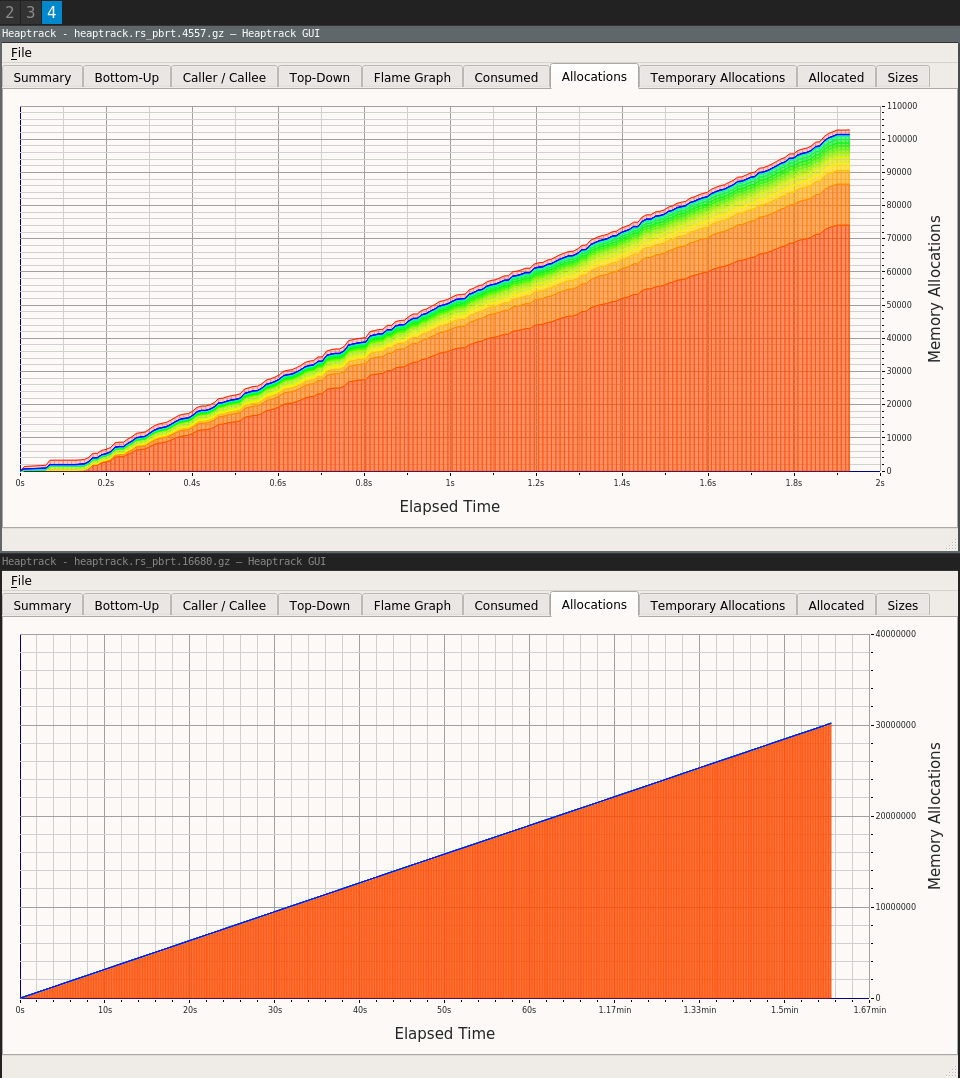## The End

I hope I didn't forget anything important. Have fun and enjoy the v0.8.2 release.